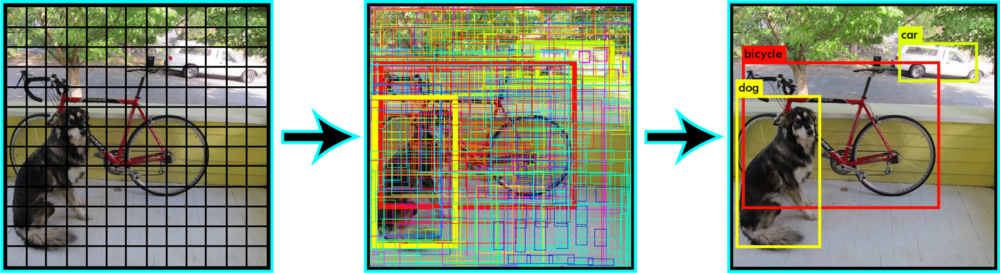What is YOLO v2 (aka YOLO 9000)?

# What is YOLO v2 (aka YOLO 9000)?

“YOLO” or “You Only Look Once”, is a massive Convolutional Neural network for object detection and classification. YOLO9000 is high speed, real-time object detection algorithm that can detect on over 9000 object categories. YOLO was originally written in a framework called darknet. Darknet is an open-source deep learning framework written in C and CUDA. It is fast, easy to install, and supports both CPU and GPU computation. If you want to know more follow this link. And, Follow this GitHub link for the translated version of darknet to TensorFlow. As mentioned in this blog article “The network divides the image into regions and predicts bounding boxes and probabilities for each region. These bounding boxes are weighted by the predicted probabilities”.

The network divides the image into regions and predicts bounding boxes and probabilities for each region. These bounding boxes are weighted by the predicted probabilities.Here is the tutorial of YOLO for real-time object detection.

See the summary of the paper You only look once: unified, Real-Time object detection paper.